Courses

# Dynamic Programming, P & NP Concept ( Advance Level) - 1

## 5 Questions MCQ Test Question Bank for GATE Computer Science Engineering | Dynamic Programming, P & NP Concept ( Advance Level) - 1

Description
This mock test of Dynamic Programming, P & NP Concept ( Advance Level) - 1 for Computer Science Engineering (CSE) helps you for every Computer Science Engineering (CSE) entrance exam. This contains 5 Multiple Choice Questions for Computer Science Engineering (CSE) Dynamic Programming, P & NP Concept ( Advance Level) - 1 (mcq) to study with solutions a complete question bank. The solved questions answers in this Dynamic Programming, P & NP Concept ( Advance Level) - 1 quiz give you a good mix of easy questions and tough questions. Computer Science Engineering (CSE) students definitely take this Dynamic Programming, P & NP Concept ( Advance Level) - 1 exercise for a better result in the exam. You can find other Dynamic Programming, P & NP Concept ( Advance Level) - 1 extra questions, long questions & short questions for Computer Science Engineering (CSE) on EduRev as well by searching above.
QUESTION: 1

### What is the time complexity of Bellman-Ford single-source shortest path algorithm on a complete graph of n vertices?

Solution:

In a complete graph total no of edges is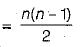Time complexity of Bellman-Ford algo for a graph having n vertices and m edges = O(nm) for a complete graph time complexity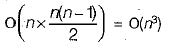QUESTION: 2

### The Ackermann’s function

Solution:

Ackermann’s function has exponential time complexity,

QUESTION: 3

### Which of the following statements are TRUE? 1. The problem of determining whether there exists a cycle in an undirected graph is in P. 2. The problem of determining whether there , exists a cycle in an undirected graph is in NP. 3. If a problem A is NP-Complete, there exists a non-deterministic polynomial time algorithm to solve A

Solution:

1. By depth first search we can find whether there exits a cycle in an undirected graph in O(n2) times so it is P problem.
2. As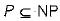so this problem will also be considered as NP problem.
3. This is the definition of NP-complete so it is trivially true.

QUESTION: 4

Consider two decision problems Q1, Q2 such that Q1 reduces in polynomial time to 3-SAT and 3-SAT reduces in polynomial time to Q2. Then which one of the following is consistent with the above statement?

Solution:

Given Q1 ≤ 3-SAT and 3-SAT ≤ Q2 3-SAT is NP- complete.
∴ It is NP as well as NP-hard.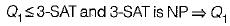is NP-problem   3-SAT ≤ Qand 3-SAT is NP-hard ⇒ Q2 is NP- hard problem.
So the strongest statements is Q1 is in NP and Q2 is NP-hard.

QUESTION: 5

Language L1 is polynomial time reducible to language L2. Language L3 is polynomial time reducible to L2, which in turn is polynomial time reducible to language L4. Which of the following is/are True?

I. If L4 ∈ P , L2 ∈ P
II If L, ∈ P or L3e P, then L2∈ P
III. L, ∈ P, if and only if L3∈ P
IV. If L4 ∈ P, then L1 ∈ P and L3 ∈ P

Solution: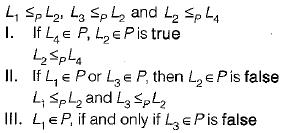if L1 ∈ P we cannot say anything about L2 from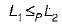Since we cannot say anything about L2 therefore we cannot say anything about L3 either from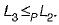So Statement III is false.
IV. If L4∈ P, then L1 ∈ P and L3 ∈ P is true.
If L4 ∈ P then from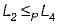we can get that L2∈ P.
and fromwe can now get that  L3 ∈ P
also fromwe can get that L1 ∈ P
So Statement IV is true.